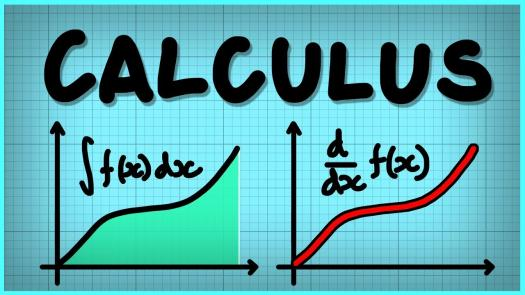# AP Calculus Test For High School!

10 Questions | Total Attempts: 109SettingsAdvanced Placement Calculus is offered by almost all high school students in the world. To many it is mathematics (well it is) but it got some intricacies that makes the calculation either complex for the not so brilliant pupils and intriguingly fun packed for the brilliant ones. If you think you're in high school and you really good with AP Calculus, test your skills with this fun packed quiz. Enjoy!

• 1.
If a = –1/b, b = –1/c, c = –1/d, d = –1/4, what is the value of a?
• A.

-4

• B.

-1/4

• C.

4

• D.

0

• 2.
When 23 is divide by 3, the remainder is b. What is the remainder when 23 is divided by 2b?
• A.

1

• B.

5

• C.

3

• D.

4

• 3.
If f(x) = x² + 108/9 and f(3b) = –7b, then what is the product of all possible real values of b?
• A.

12

• B.

13

• C.

15

• D.

18

• 4.
If y² – z² = 19 and y – z = 7, what is the value of y?
• A.

12

• B.

5

• C.

18

• D.

0

• 5.
If 12(10v + 8)(6v + 4)(2v) = 0, then how many different possible values of v exist?
• A.

1

• B.

4

• C.

3

• D.

5

• 6.
What is the remainder when the sum of three consecutive even integers is divided by 6?
• A.

9

• B.

0

• C.

7

• D.

5

• 7.
If v = –2, then v + v² - v³ + v⁴  - v^5 =
• A.

44

• B.

67

• C.

58

• D.

76

• 8.
If 1/8 + 1/10 = v/y, and v and y are positive integers and v/y is in its simplest reduced form, what is the value of v?
• A.

9

• B.

18

• C.

27

• D.

36

• 9.
If [vz/2]² = 1 v^z = 1, where v and z are greater than zero, what is the value of z?
• A.

5

• B.

4

• C.

3

• D.

2

• 10.
If 4b • c² = 4b+1 • c and b and c are both positive integers, what is the value of c?
• A.

12

• B.

13

• C.

14

• D.

15

Related TopicsBack to top